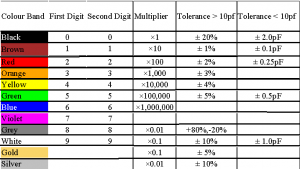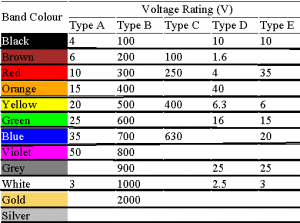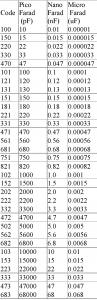Home > electronic components > capacitors > colour coding in capacitors

Colour Coding in Capacitors

Similar to resistors the colour coding system is also used in the capacitors. Using this international colour coding system the user can determine the value of capacitance of the capacitor including the tolerances. In this colour coding system the colour bands are used to determine the capacitance value. Table bellow shows the colour bands to determine the value of the capacitor.Similar to colour code to determine the capacitor value, the colour bands are also used to determine the voltage rating of the capacitor. The colour bands used to determine the voltage rating of the capacitor are shown in below table.Here, the various types used in voltage rating are,
• Type A – Dipped Tantalum Capacitors.
• Type B – Mica Capacitors.
• Type C – Polyester/Polystyrene Capacitors.
• Type D – Electrolytic 4 Band Capacitors.
• Type E – Electrolytic 3 Band Capacitors.
Normally the values of capacitance are written over the capacitors. The example of this capacitors are follows,
1) n25= 0.25 nano Farad
2) 2n5= 2.5 nano Farad
3) 25n= 25 nano Farad
4) 1K= 1000 pico Farad
5)10K= 10× 1000 pico Farad
6) 100K= 100× 1000 pico Farad
Normally the capacitor code consists of 2 or 3 numbers and the tolerance code. The first two digits are used to determine the value of the capacitor in pico Farads. Third digit in the capacitor is used as a multiplier in the power of ten. The examples of this are as follows,
1) 251=25×10 pico Farad Hence 250 pico Farad
Table below shows the codes written on the capacitors and the determined value of the capacitance.